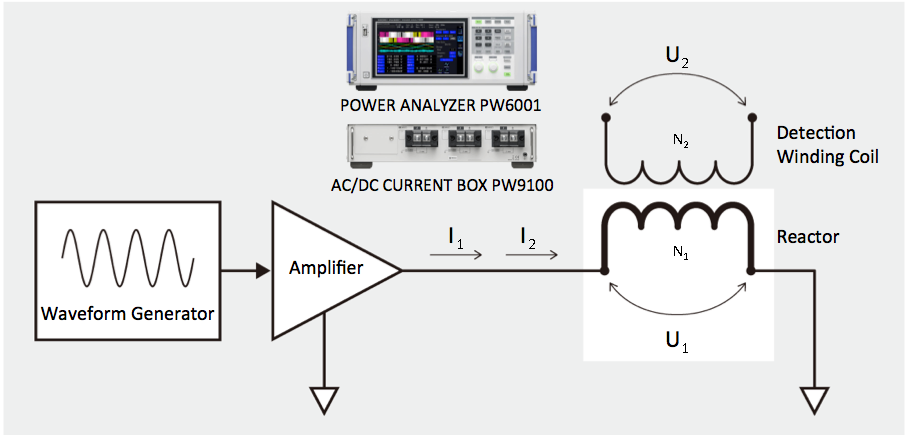# Loss Analysis of Reactors While in Operation

## Why Should Reactor Loss be Analyzed?

By directly measuring reactor loss, as well as iron loss and copper loss ratios within reactor loss, you can significantly reduce research and development time and identify areas of improvement for reaching even higher efficiency and smaller form factors.
• High iron loss ->  Issues with the core likely; focus on improving the structure and materials.
• High copper loss ->  Issues with the winding coil likely; focus on improving the winding method or materials.

## How is Reactor Loss Measured?

High frequency current is applied to the reactor.  The Power Analyzer then measures the reactor loss, as well as core loss using a detection winding coil that can identify core magnetic flux.

## Measureable Parameters

•  Reactor “Total Loss” is equal to power calculated from U1 and I1.
•  Reactor “Iron Loss (Core Loss)” is equal to power calculated from U2 and I2. (“Iron Loss” is loss generated from current magnetization of the core magnetic material.)
•  “Copper Loss” can be derived from “Total Loss” and “Iron Loss”.  (“Cooper Loss” is loss generated from the resistance component of the winding coil.)
•  “Iron Loss Ratio” and “Copper Loss Ratio” can be derived from “Iron Loss” and “Copper Loss.”## Key Benefits of Power Analyzers

Thanks to the wide frequency bandwidth and phase shift correction function, devices that exhibit high frequency and lower power factor power such as reactors and capacitors can be accurately measured. Based on your own defined formulas, compute and display the parameters required for evaluation directly on the power analyzer.  With the high speed simultaneous analytical capabilities available on the motor analysis engine, easily verify waveforms, calculated values, harmonics, noise, FFT and other important parameters on the toggle display.